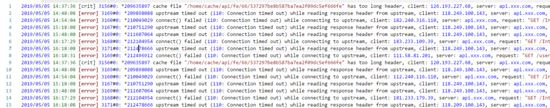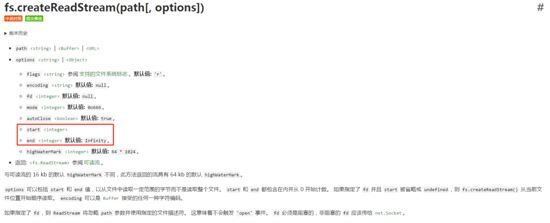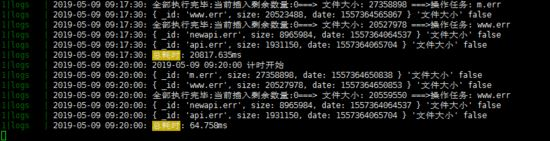# NodeJS读取分析Nginx错误日志的方法

更新时间：2019年05月14日 14:35:07   作者：zpzxgcr我要评论这里我们用到readline

• 文件逐行读取：比如说进行日志分析。
• 自动完成：比如输入npm，自动提示"help init install"。
• 命令行工具：比如npm init这种问答式的脚手架工具。 这里我们主要做日志分析其他的感兴趣可以琢磨一下

```const readline = require('readline');
const fs = require('fs');
const path = require('path');
console.time('readline-time')
const rl = readline.createInterface({
input: fs.createReadStream(path.join(__dirname, '../public/api.err.log'), {
start: 0,
end: Infinity
}),

});
let count = 0;
rl.on('line', (line) => {
const arr = line.split(', ');
const time = arr.split('*').split('[').replace(/\//g, '-');//获取到时间
const error = arr.split('*').split(/\d\s/);//错误原因
const client = arr.split(' ');//请求的客户端
const server = arr.split(' ');//请求的网址
const url = arr.match(/\s\/(\S*)\s/).trim()//获取请求链接
const upstream = arr.match(/(?<=").*?(?=")/g);//获取上游
const host = arr.match(/(?<=").*?(?=")/g);//获取host
const referrer = arr ? arr.match(/(?<=").*?(?=")/g) : '';//来源
console.log(`时间:\${time}-原因:\${error}-客户端:\${client}-网址:\${server}-地址:\${url}-上游:\${upstream}-主机:\${host}-来源:\${referrer}`);
count++;
});
rl.on('close', () => {
let size = fs.statSync(path.join(__dirname, '../public/api.err.log')).size;
console.log(`读取完毕:\${count};文件位置:\${size % 2 === 0}`);
console.timeEnd('readline-time')
});````let size = fs.statSync(path.join(__dirname, '../public/api.err.log')).size;````rl.on('close', async () => {
let count = 0;
for (let i of rlist) {
count++;
if (count % 500 === 0) {
const res = await global.db.collection('logs').bulkWrite(rlist.slice(count, count + 500), { ordered: false, w: 1 }).catch(err => { console.error(`批量插入出错\${err}`) });
} else if (count === rlist.length - 1) {
//批量插入 数据
const res = await global.db.collection('logs').bulkWrite(rlist.slice(rlist - (rlist % 500), rlist.length), { ordered: false, w: 1 });
let size = fs.statSync(addres).size;
size = size % 2 === 0 ? size : size + 1;//保证字节大小是偶数 不然会出现读取上行内容不完整的情况
count = 0;
rlist.length = [];
//更新数据库里面文件的size
global.db.collection('tasks').updateOne({ _id: addre }, { \$set: { _id: addre, size, date: +new Date() } }, { upsert: true });
}
}
resolve(true);
})```

```if (typeof s === 'string' && s) {
var lines = s.split(/\r\n|\n|\r/);
for (var i = 0, len = lines.length; i < len; i++) {
if (i > 0) {
this._line();
}
this._insertString(lines[i]);
}
}
...
Interface.prototype._line = function() {
const line = this._addHistory();
this.clearLine();
this._onLine(line);
};
...
Interface.prototype._onLine = function(line) {
if (this._questionCallback) {
var cb = this._questionCallback;
this._questionCallback = null;
this.setPrompt(this._oldPrompt);
cb(line);
} else {
this.emit('line', line);
}
};```

```db.logs.aggregate(
// Pipeline
[
// Stage 1
{
\$group: {
'_id': { 'client': '114.112.163.28', 'server': '\$server', 'error': '\$error', 'url': '\$url', 'upstream': '\$upstream','date':'\$date' ,'msg':'\$msg' } ,

'date':{'\$addToSet':'\$date'},
count: { '\$sum': 1 }
}
},
// Stage 2
{
\$match: {
count: { \$gte: 1 },
date: ['2019-05-10']

}
},
{
\$sort: {
count: -1
}
},
],
// Options
{
cursor: {
batchSize: 50
},
allowDiskUse: true
}
);```